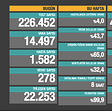# Statcast Data Visualization in R

`FB_over_95 <- NL_CY %>%  filter(pitch_type == "FF") %>%  group_by(player_name) %>%  summarize('95+' = sum(release_speed > 95, na.rm = TRUE))`
`ggplot(FB_over_95, aes(x = player_name, y = `95+`)) +   geom_bar(stat = "identity")`
`ggplot(FB_over_95, aes(x = reorder(player_name, -`95+`), y = `95+`, fill = player_name)) +   geom_bar(stat = "identity") +  labs(x = "Pitcher", y = "", title = "Number of fastballs over 95 MPH") +  theme_bw() +  theme(legend.position = "none")`
`Fastballs_deGrom <- NL_CY %>%  filter(player_name == "Jacob deGrom", pitch_type == "FF")ggplot(Fastballs_deGrom, aes(x = release_speed)) +  geom_density()ggplot(Fastballs_deGrom, aes(x = release_speed)) +   geom_density(color = "red", fill = "lightblue") +  labs(x = "Velocity (MPH)", title = "Distribution of Fastball Velocity") +  theme_bw()`
`Fastballs_NL_CY <- NL_CY %>%  filter(pitch_type == "FF")ggplot(Fastballs_NL_CY, aes(x = player_name, y = release_speed)) +  geom_boxplot()ggplot(Fastballs_NL_CY, aes(x = player_name, y = release_speed, fill = player_name)) +  geom_boxplot(width = 0.5) +   labs(x = "Pitcher", y = "Velocity (MPH)", title = "Distribution of Fastball Velocity") +  theme_bw() +  theme(legend.position = "none")`
`ggplot(NL_ROY, aes(x = release_speed, y = release_spin_rate, color = pitch_type)) +  geom_point()ggplot(NL_ROY, aes(x = release_speed, y = release_spin_rate, color = pitch_type)) +  geom_point(size = 2, na.rm = TRUE) +  scale_x_continuous(limits = c(80,100), breaks = c(80,85,90,95,100)) +  scale_y_continuous(limits = c(2000,3250), breaks = c(2000,2250,2500,2750,3000,3250)) +  labs(x = "Velocity (MPH)", y = "Spin Rate (RPM)", color = "Pitch Type",       title = "Devin Williams Velocity vs. Spin Rate") +  theme_bw() +  theme(legend.position = "bottom")`
`cor_df <- statcast_data %>%  filter(pitch_type == "FF") %>%  group_by(player_name, pitch_type) %>%  summarize('n' = n(),            'mean_release_speed' = mean(release_speed, na.rm = TRUE),            'sw_str_percentage' = sum(description %in% c("swinging_strike", "swinging_strike_blocked"))/n()) %>%  filter(n > 100, mean_release_speed > 85)ggscatter(data = cor_df, x = "mean_release_speed", y = "sw_str_percentage",           add = "reg.line", cor.coef = TRUE, cor.method = "pearson",          conf.int = TRUE, add.params = list(color = "red", fill = "lightgray"),          xlab = "Velocity (MPH)", ylab = "Swinging Strike %",           title = "Correlation: AVG FB Velocity vs. Sw. Strike %")`
`NL_CY\$game_date <- as.Date(NL_CY\$game_date)time_df <- NL_CY %>%  filter(pitch_type == "FF") %>%  group_by(player_name, pitch_type, game_date) %>%  summarize('n' = n(),            'mean_release_speed' = mean(release_speed, na.rm = TRUE))ggplot(data = time_df, aes(x = game_date, y = mean_release_speed, group = player_name)) +   geom_line() + geom_point()ggplot(data = time_df, aes(x = game_date, y = mean_release_speed, group = player_name, color = player_name)) +   geom_line(size = 1) + geom_point(size = 3) +  labs(y = "Velocity (MPH)", title = "Average FB Velocity by Game", color = "") +  theme_bw() +  theme(legend.position = "bottom", axis.title.x = element_blank())`

--

--

--

## More from Sam Bornstein

Driveline Baseball Operations Analyst

Love podcasts or audiobooks? Learn on the go with our new app.

## Recommended from Medium## A Comprehensive Guide for Classes in Python## Information Design; Data Visualization## Model interpretability — Making your model confesses: Shapley values## Analyzing K-Pop Using Machine Learning | Part 2— Exploratory Data Analysis (EDA)## Elegran Insights: Weekly Manhattan & Brooklyn Market## Big Thoughts on Big Data## Sam Bornstein

Driveline Baseball Operations Analyst

## Mass Data Reconciliation Made Simple by R## The Data Sandbox | Creating Dashboards in R